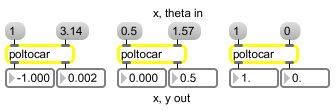# poltocar

Polar to Cartesian coordinate conversion

## Description

poltocar takes a polar-coordinate pair consisting of distance and angle values and converts them into a cartesian-coordinate pair consisting of real and imaginary values.

None.

## Messages

 bang Causes poltocar to output the very last conversion it has calculated. int polar-coordinate [int] Converted to float. float polar-coordinate [float] In left inlet: The distance portion of a polar coordinate pair to be converted into a Cartesian coordinate pair consisting of real and imaginary values. When used in an audio context, the value represents magnitude (amplitude) portion of a polar coordinate pair to be converted into a cartesian (real/imaginary) coordinate pair. In right inlet: The angle portion of a polar coordinate pair to be converted into a Cartesian coordinate pair consisting of real and imaginary values. When used in an audio context, the value represents the phase portion of a polar coordinate pair to be converted into a cartesian (real/imaginary) coordinate pair.

## Output

float: Out left outlet: The real portion of a Cartesian coordinate pair.

Out right outlet: The imaginary portion of a Cartesian coordinate pair.

## ExamplesConvert Polar to Cartesian coordinates